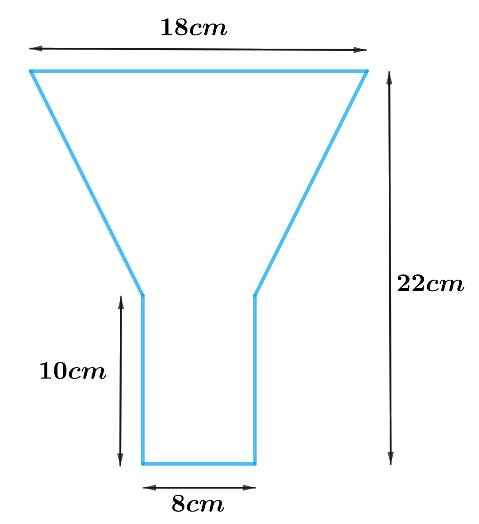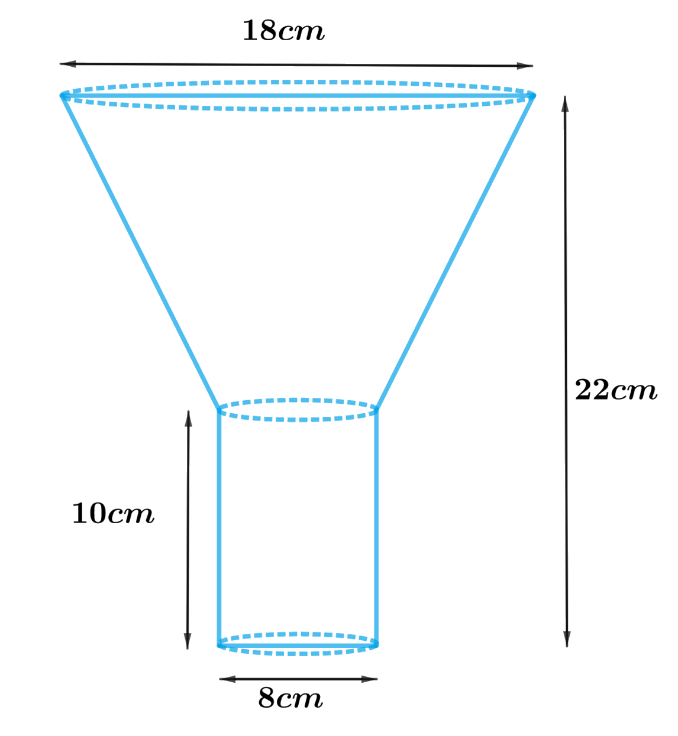# Ex.13.5 Q5 Surface Areas and Volumes Solution - NCERT Maths Class 10

Go back to  'Ex.13.5'

## Question

An oil funnel made of tin sheet consists of a $$10\,\rm{cm}$$ long cylindrical portion attached to a frustum of a cone. If the total height is $$22 \,\rm{cm,}$$ diameter of the cylindrical portion is $$8\rm{cm}$$ and the diameter of the top of the funnel is $$18\,\rm{cm,}$$ find the area of the tin sheet required to make the funnel (see Fig. 13.25).Video Solution
Surface Areas And Volumes
Ex 13.5 | Question 5

## Text Solution

What is Known?

Length of the cylindrical part is $$10\,\rm{ cm}$$ and diameter is $$8 \,\rm{cm}$$. Diameter of top of the funnel is $$18\, \rm{cm}$$ and total height of funnel is $$22\,\rm{ cm.}$$

What is Unknown?

Area of tin sheets required to make the funnel

Reasoning:Since the funnel is open at the top and the bottom. Hence, open at the junction of the frustum of cone and cylindrical part.

Therefore,

Area of tin sheet required to make the funnel $$=$$ CSA of frustum of cone $$+$$ CSA of the cylinder

We will find the CSA of the frustum by using formulae;

CSA of frustum of a cone $$= \pi \left( {{r_1} + {r_2}} \right)l$$

Slant height, $$l = \sqrt {{h^2} + {{\left( {{r_1} - {r_2}} \right)}^2}}$$

where $$r1, r2, h$$ and $$l$$ are the radii height and slant height of the frustum of the cone respectively.

CSA of the cylinder $$= 2\pi rh$$

where $$r$$ and $$h$$ are radius and height of the cylinder respectively.

Steps:

Height of the funnel, $$H = 22cm$$

Height of cylindrical part, $$h = 10cm$$

Height of frustum of cone, $${h_1} = 22cm - 10cm = 12cm$$

Radius of top part of frustum of cone, \begin{align}{r_1} = \frac{{18cm}}{2} = 9cm\end{align}

Radius of lower part of frustum of cone, \begin{align}{r_2} = \frac{{8cm}}{2} = 4cm\end{align}

Radius of cylindrical part, $${r_2} = 4cm$$

Slant height of frustum, \begin{align}l = \sqrt {{h_1}^2 + {{\left( {{r_1} - {r_2}} \right)}^2}}\end{align}

\begin{align}&= \sqrt {{{\left( {12cm} \right)}^2} + {{\left( {9cm - 4cm} \right)}^2}} \\&= \sqrt {144c{m^2} + 25c{m^2}} \\&= \sqrt {169c{m^2}} \\&= 13cm\end{align}

Area of tin sheet required to make the funnel $$=$$ CSA of frustum of cone $$+$$ CSA of the cylinder

\begin{align} & =\pi \left( {{r}_{1}}+{{r}_{2}} \right)l+2\pi {{r}_{2}}h \\ & =\pi \left[ \left( {{r}_{1}}+{{r}_{2}} \right)l+2{{r}_{2}}h \right] \\ & =\frac{22}{7}\left[ \begin{array} & \left( 9cm+4cm \right)\times 13cm+ \\ 2\times 4cm\times 10cm \\ \end{array} \right] \\ & =\frac{22}{7}\left[ 169c{{m}^{2}}+80c{{m}^{2}} \right] \\ & =\frac{22}{7}\times 249c{{m}^{2}} \\ & =\frac{5478}{7}c{{m}^{2}} \\ & =782\frac{4}{7}c{{m}^{2}} \\ \end{align}

Learn from the best math teachers and top your exams

• Live one on one classroom and doubt clearing
• Practice worksheets in and after class for conceptual clarity
• Personalized curriculum to keep up with school